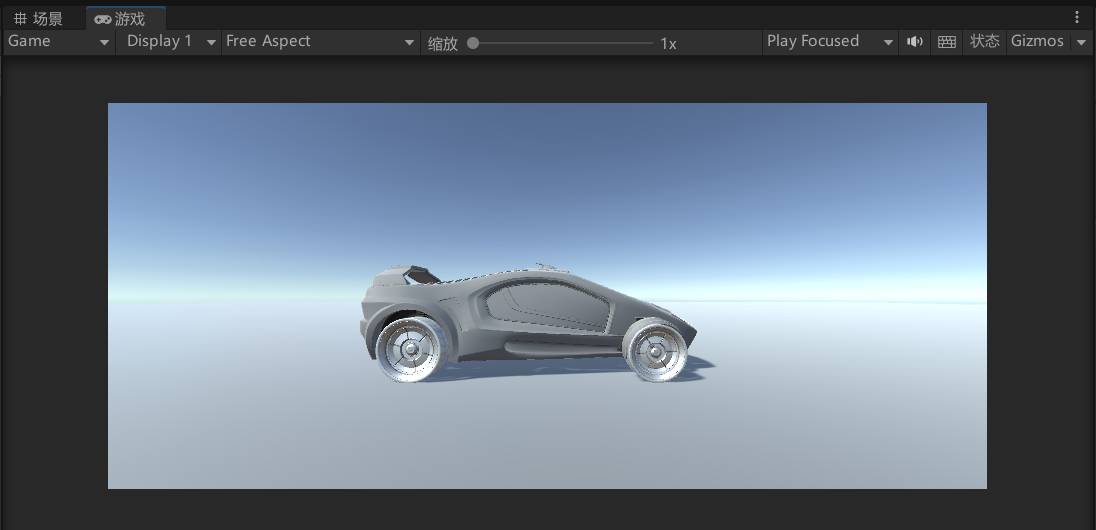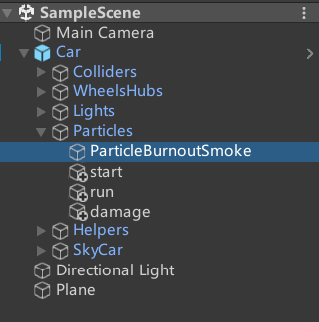# Unity3d粒子系统 汽车尾气模拟

## 场景布置

Standard Assets.unitypackage（68.4M）

https://blog.csdn.net/Sakuya__/article/details/113815974## 粒子效果模拟``````public class CarStatus : MonoBehaviour
{
// 定义需要切换的粒子效果
public ParticleSystem[] particleSystems;
// 当前粒子效果的索引
public int currentIndex = 0;
void Start()
{
particleSystems = new ParticleSystem;
particleSystems = transform.Find("Particles/ParticleBurnoutSmoke").GetComponent<ParticleSystem>();
particleSystems = transform.Find("Particles/start").GetComponent<ParticleSystem>();
particleSystems = transform.Find("Particles/run").GetComponent<ParticleSystem>();
particleSystems = transform.Find("Particles/damage").GetComponent<ParticleSystem>();
// 将所有粒子效果关闭
for (int i = 0; i < particleSystems.Length; i++)
{
particleSystems[i].Stop();
}
}

void Update()
{
// 当按下键盘对应数字键时，切换粒子效果
if (Input.GetKeyDown(KeyCode.Alpha1))
{
// 将当前粒子效果关闭
particleSystems[currentIndex].Stop();
// 将当前粒子效果索引设置为0
currentIndex = 0;
// 将当前粒子效果打开
particleSystems[currentIndex].Play();
}
else if (Input.GetKeyDown(KeyCode.Alpha2))
{
particleSystems[currentIndex].Stop();
currentIndex = 1;
particleSystems[currentIndex].Play();
}
else if (Input.GetKeyDown(KeyCode.Alpha3))
{
particleSystems[currentIndex].Stop();
currentIndex = 2;
particleSystems[currentIndex].Play();
}
else if (Input.GetKeyDown(KeyCode.Alpha4))
{
particleSystems[currentIndex].Stop();
currentIndex = 3;
particleSystems[currentIndex].Play();
}
}
}``````

``````public class CameraMove : MonoBehaviour
{
public GameObject follow;  // 跟随的对象
public float speed = 8f;  // 相机跟随的速度
Vector3 offset;  // 相机与物体相对偏移位置

void Start()
{
offset = transform.position - follow.transform.position;
}

void FixedUpdate()
{
Vector3 target = follow.transform.position + offset;  // 计算目标位置
transform.position = Vector3.Lerp(transform.position, target, speed * Time.deltaTime);  // 摄像机自身位置到目标位置平滑过渡
}
}``````

## 粒子效果展示

https://www.yizuodi.cn/category/3DGame/